## Monday, 21 May 2012

### Unit 1 Expressions and formulae

Outcome 1.1
Applying numerical skills to simplify surds/expressions using the law of indices.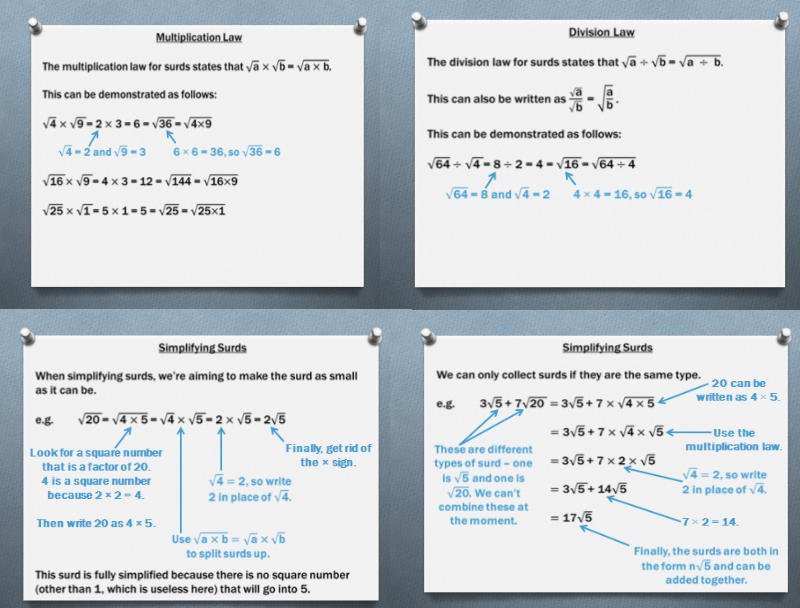Surds Overview

### Surds - Rationalising the denominator (BBC)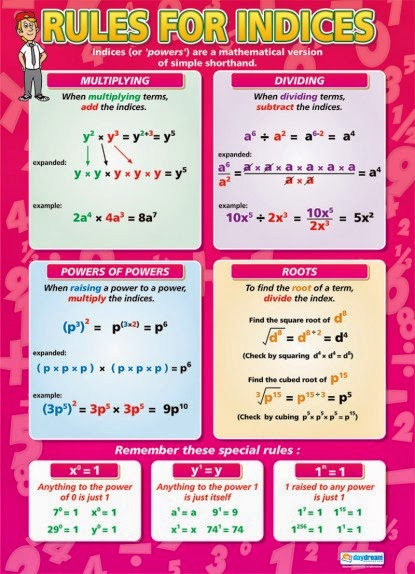Rules for indices

## Test - surds and indices (BBC)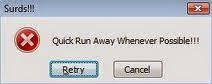Outcome 1.2
Applying algebraic skills to manipulate expressions.Removing Brackets

## Test  - Expansion of Brackets (BBC)Factorising - Highest Common Factor

### Factorising - highest common factor (BBC)Difference of two Squares

### Factorising - difference of two squares (BBC)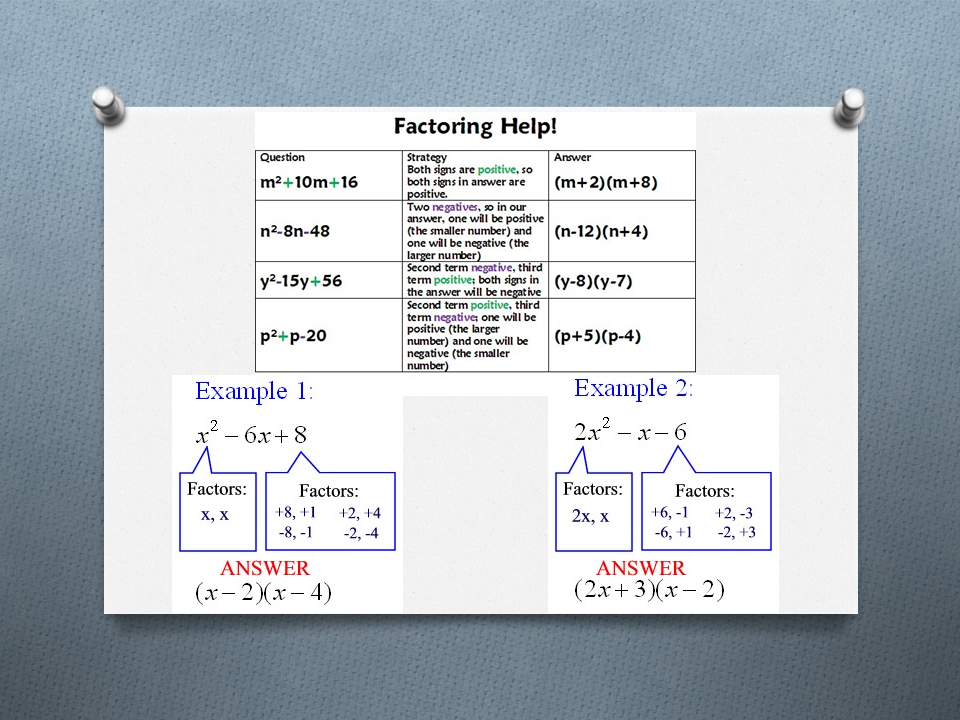Factorising Trinomials

### Factorising trinomials where coefficient of x^2 is greater than 1 (BBC)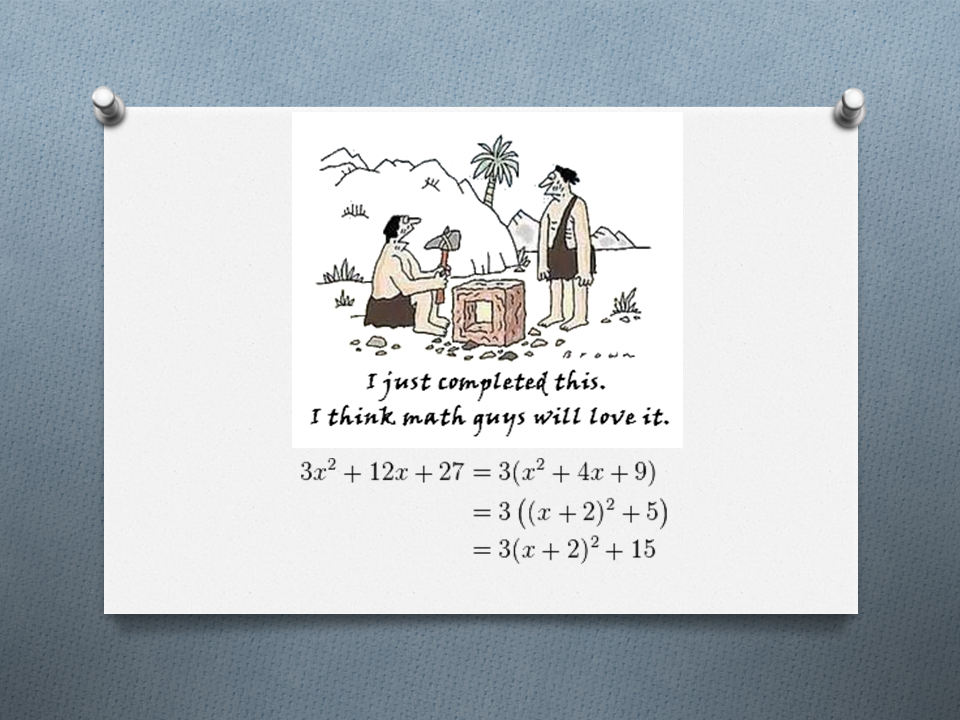Completing the square

## Test - Factorising (BBC)

Outcome 1.3
Applying algebraic skills to algebraic fractions.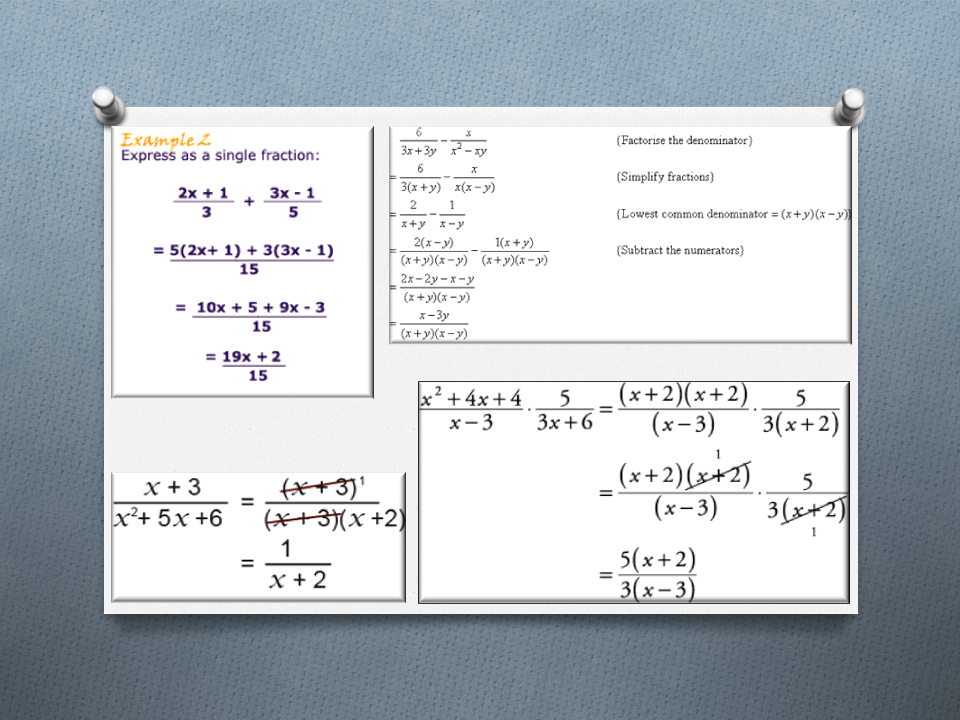Algebraic fractions

## Test - Algebraic fractions (BBC)

Outcome 1.4
Applying geometric skills linked to the use of formulae.Gradient of a straight line

### Gradient of a straight line (BBC)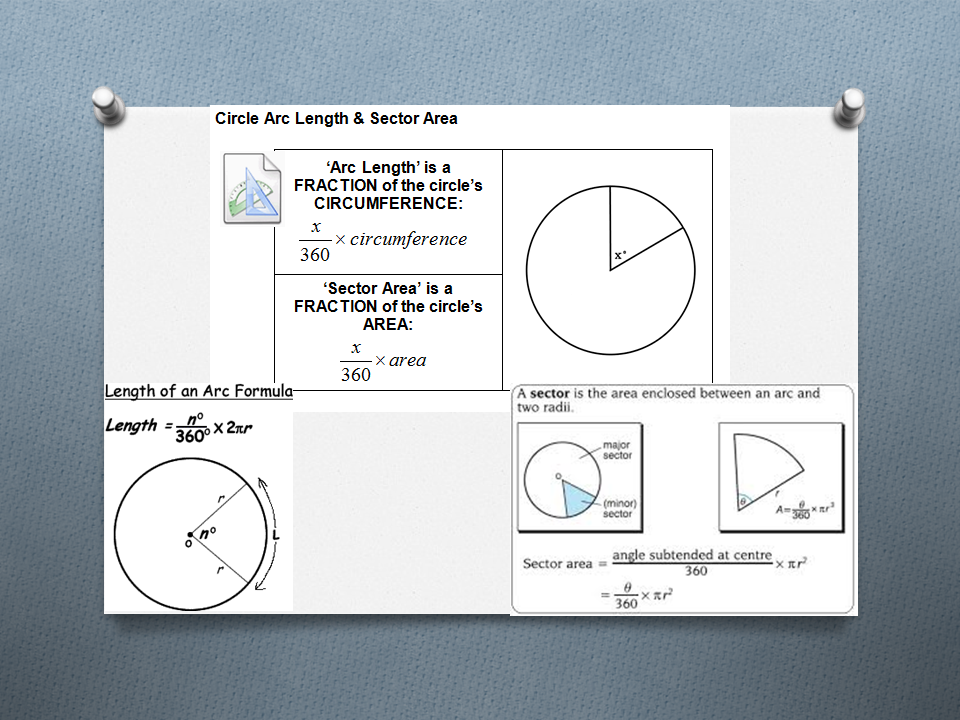Arc length and sector area

## Test - Circle geometry (BBC)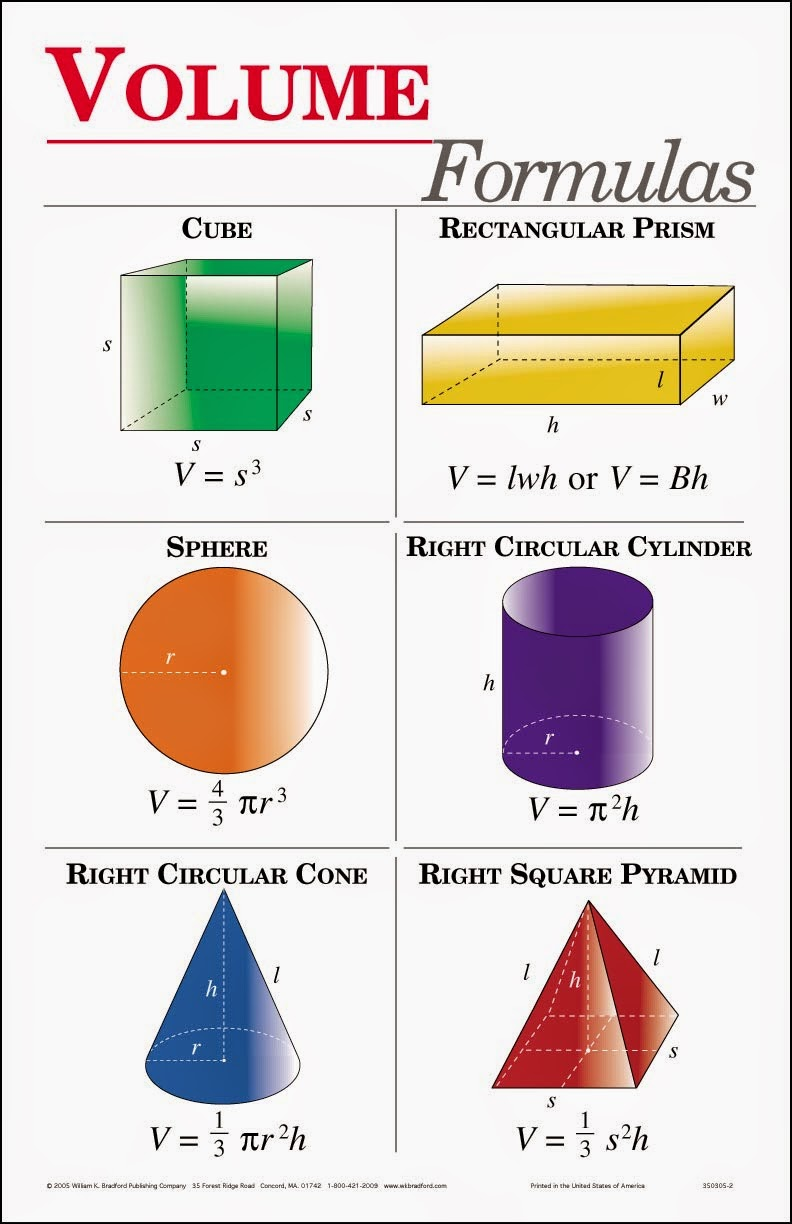Volume of shapes

## Test - Volume (BBC)Rounding to significant figures